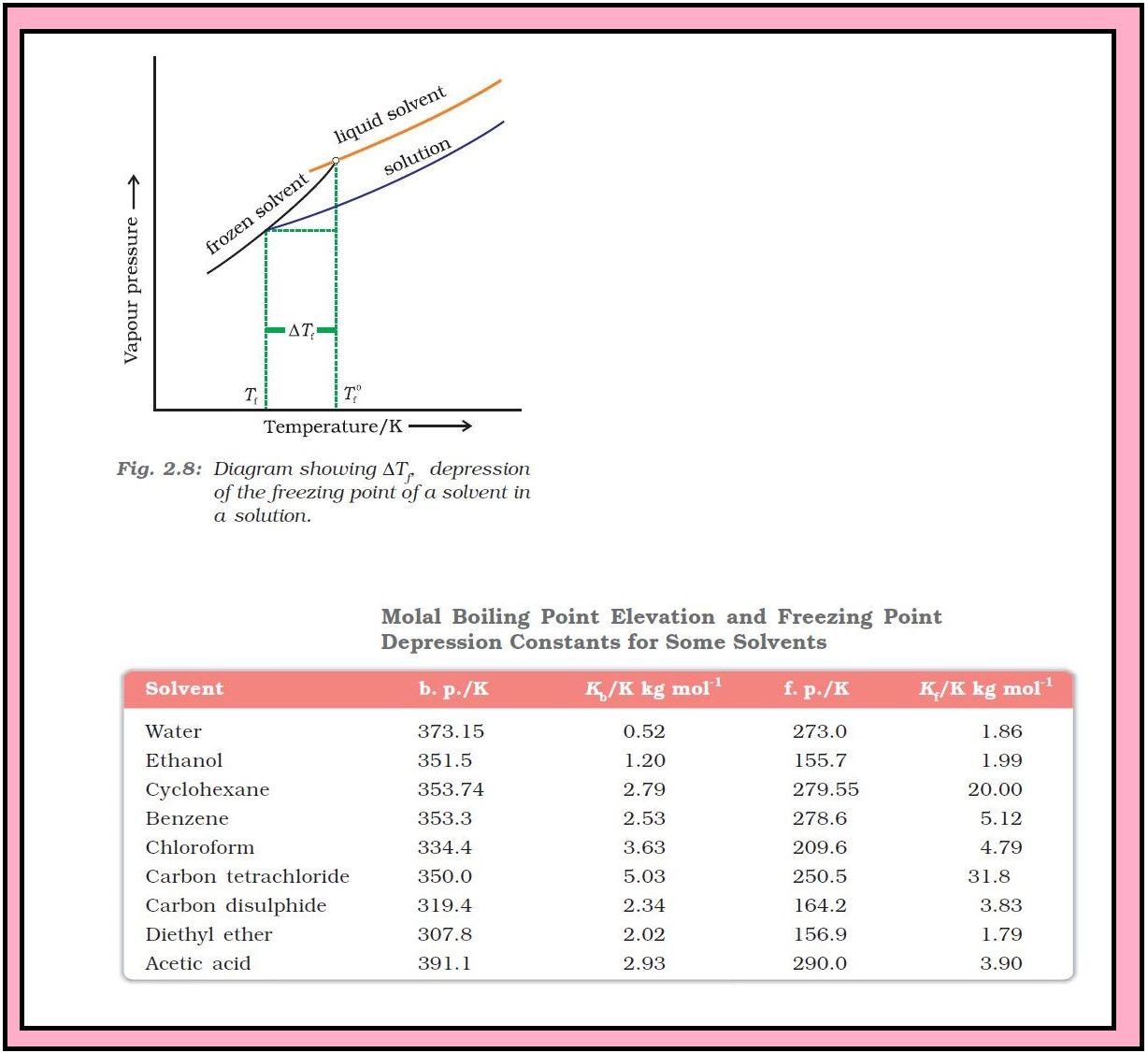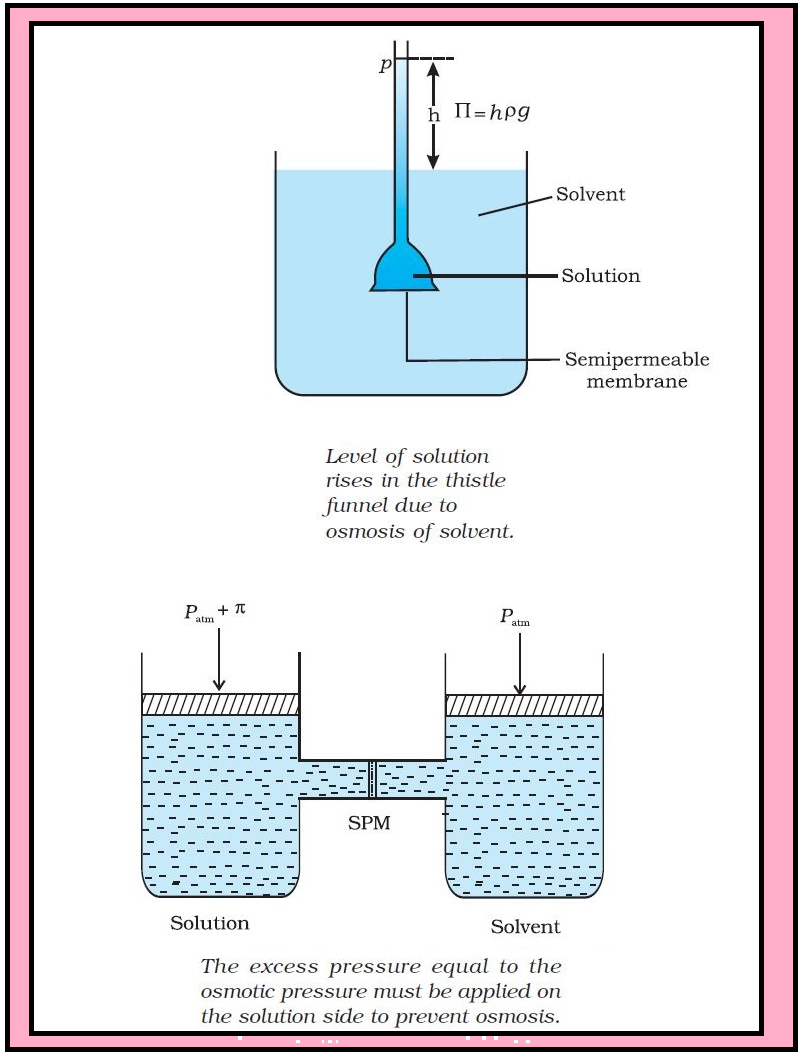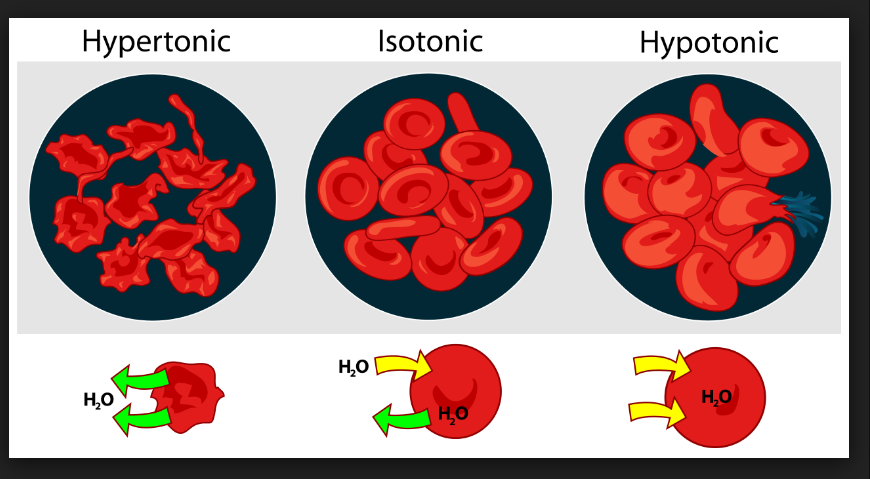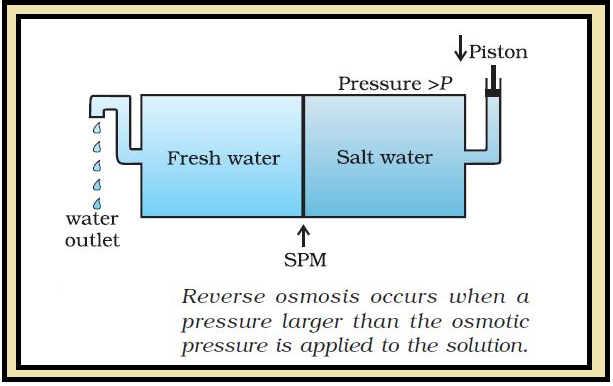Chemistry Depression of Freezing Point and Osmotic Pressure
Click for Only Video

### Topics Covered :

● Depression of Freezing Point
● Osmosis
● Osmotic Pressure
● Isotonic Solutions
● Reverse Osmosis

### Depression of Freezing Point :=> The lowering of vapour pressure of a solution causes a lowering of the freezing point compared to that of the pure solvent.

=> At the freezing point of a substance, the solid phase is in dynamic equilibrium with the liquid phase.

color{green}("Definition") : The freezing point of a substance may be defined as the temperature at which the vapour pressure of the substance in its liquid phase is equal to its vapour pressure in the solid phase.

=> A solution freezes when its vapour pressure equals the vapour pressure of the pure solid solvent as it is clear from Fig.

=> According to Raoult’s law, when a non-volatile solid is added to the solvent its vapour pressure decreases and now it would become equal to that of solid solvent at lower temperature. Therefore, the freezing point of the solvent decreases.

Let T_f^0 be the freezing point of pure solvent and T_f be its freezing point when non-volatile solute is dissolved in it.

The decrease in freezing point, DeltaT_f = T_f^0 - T_f is known as depression in freezing point.

Depression of freezing point (ΔT_f) for dilute solution (ideal solution) is directly proportional to molality, m of the solution. Thus,

DeltaT_f prop m or color{red}(DeltaT_f = K_f m) ................(1).

The proportionality constant, K_f, which depends on the nature of the solvent is known as Freezing Point Depression Constant or Molal Depression Constant or Cryoscopic Constant.

● The unit of K_f is K kg mol^(-1).

● Values of K_f for some common solvents are listed in Table.

If w_2 gram of the solute having molar mass as M_2, present in w_1 gram of solvent, produces the depression in freezing point ΔT_f of the solvent then molality of the solute is given by the equation.

color{red}(m = ( w_2/M_2)/(w_1/1000))

Substituting this value of molality we get:

color{red}(DeltaT_f = (K_f xx w_2/M_2)/(M_2 xx w_1)) .......................(2).

color{red}(DeltaT_f = (K_f xx w_2 xx 1000)/(DeltaT_f xx w_1)) .....................(3).

Thus for determining the molar mass of the solute we should know the quantities w_1, w_2, ΔT_f, along with the molal freezing point depression constant.

=> Value of K_f is given as :

color{red}(K_f = (R xx M_1 xx T_f^2)/(1000 xx Delta_text(fus) H)) ................(4).

Here the symbols R and M_1 stand for the gas constant and molar mass of the solvent respectively and T_f denote the freezing point of the pure solvent in kelvin.

Further, Δ_text(fus)H represents the enthalpy for the fusion of the solvent.

Q 290655647845 g of ethylene glycol (C_2H_6O_2) is mixed with 600 g of water. Calculate (a) the freezing point depression and (b) the freezing point of the solution.Solution:

Depression in freezing point is related to the molality, therefore, the molality of the solution with respect to ethylene glycol  = text(moles of ethylene glycol)/text(mass of water in kilogram)

Moles of ethylene glycol = (45 g)/(62 g mol^(-1)) = 0.73 mol

Mass of water in kg  = (600 g)/(1000 g kg^(-1)) = 0.6 kg

Hence molality of ethylene glycol  = (0.73 mol)/(0.60 kg) = 1.2 mol kg^(-1)

Therefore freezing point depression. AT_f = 1.86 K kg mol^(-1) xx 1.2 mol kg^(-1) = 2.2K

Freezing point of the aqueous solution = 273.15 K – 2.2 K = 270.95 K
Q 29366565721.00 g of a non-electrolyte solute dissolved in 50 g of benzene lowered the freezing point of benzene by 0.40 K. The freezing point depression constant of benzene is 5.12 K kg mol^(–1). Find the molar mass of the solute.Solution:

Substituting the values of various terms involved in equation (2.36) we get M_2 = (5.12 K kg mol^(-1) xx 1.00g xx 1000 g kg^(-1))/(0.40xx50 g)

 = 256g mol^(-1)

Thus, molar mass of the solute = 256 g mol^(-1)

### Osmosis and Osmotic Pressure :There are many phenomena which we observe in nature or at home.

color{red}("Examples") : (i) Raw mangoes shrivel when pickled in brine (salt water)

(ii) Wilted flowers revive when placed in fresh water

(iii) Blood cells collapse when suspended in saline water

We find one thing common in all, that is, all these substances are bound by membranes.

color{green}("Properties of Membrane") : (i) These membranes can be of animal or vegetable origin and these occur naturally such as pig’s bladder or parchment or can be synthetic such as cellophane.

(ii) These membranes appear to be continuous sheets or films but they contain a network of submicroscopic holes or pores.

(iii) Small solvent molecules, like water, can pass through these holes but the passage of bigger molecules like solute is hindered.

(iv) These membranes are known as semipermeable membranes (SPM).

color{green}("Osmosis") : Assume that only solvent molecules can pass through these semipermeable membranes. If this membrane is placed between the solvent and solution as shown in Fig., the solvent molecules will flow through the membrane from pure solvent to the solution. This process of flow of the solvent is called osmosis. The flow will continue till the equilibrium is attained.

color{green}("Osmotic Pressure") : The flow of the solvent from its side to solution side across a semipermeable membrane can be stopped if some extra pressure is applied on the solution. This pressure that just stops the flow of solvent is called osmotic pressure of the solution.

=> The flow of solvent from dilute solution to the concentrated solution across a semipermeable membrane is due to osmosis.

color{green}("Note") : (i) Solvent molecules always flow from lower concentration to higher concentration of solution.

(ii) The osmotic pressure has been found to depend on the concentration of the solution.

Osmotic pressure is a colligative property as it depends on the number of solute molecules and not on their identity. For dilute solutions, it has been found experimentally that osmotic pressure is proportional to the molarity, C of the solution at a given temperature T. Thus :

color{red}(pi = CRT) ..............(5).

Here pi is the osmotic pressure and R is the gas constant.

color{red}(pi = ( n_2/V)RT) .................(6).

Here V is volume of a solution in litres containing n_2 moles of solute. If w_2 grams of solute, of molar mass, M_2 is present in the solution, then color{red}(n_2 = w_2 / M_2) and we can write, color{red}(pi V = (w_2 RT)/M_2) ..........(7).

or color{red}(M_2 = (w_2 RT)/(pi V)) ...................(8).

Thus, knowing the quantities w_2, T, pi and V we can calculate the molar mass of the solute.

Measurement of osmotic pressure provides another method of determining molar masses of solutes.

color{green}("Note") : This method is widely used to determine molar masses of proteins, polymers and other macromolecules.

=> The osmotic pressure method has the advantage over other methods as pressure measurement is around the room temperature and the molarity of the solution is used instead of molality.

As compared to other colligative properties, its magnitude is large even for very dilute solutions.

The technique of osmotic pressure for determination of molar mass of solutes is particularly useful for biomolecules as they are generally not stable at higher temperatures and polymers have poor solubility.
Q 2916656579200 cm^3 of an aqueous solution of a protein contains 1.26 g of the protein. The osmotic pressure of such a solution at 300 K is found to be 2.57 × 10^(-3) bar. Calculate the molar mass of the protein.Solution:

The various quantities known to us are as follows: ð = 2.57xx10^(-3) bar.

V = 200 cm^3 = 0.200 litre , T = 300 K

R = 0.083 L bar mol^(-1) K^(-1)

Substituting these values in equation (2.42) we get

M_2 = (1.26g xx 0.083 L text(bar) K^(-1) mol^(-1) xx 300 K)/(2.57xx10^(-3) text(bar) xx 0.200L) = 61.022g mol^(-1)

### Isotonic Solutions :color{green}("Definition") : Two solutions having same osmotic pressure at a given temperature are called isotonic solutions.

=> When such solutions are separated by semipermeable membrane no osmosis occurs between them.

=> For example, the osmotic pressure associated with the fluid inside the blood cell is equivalent to that of 0.9% (mass/volume) sodium chloride solution, called normal saline solution and it is safe to inject intravenously.

=> If we place the cells in a solution containing more than 0.9% (mass/volume) sodium chloride, water will flow out of the cells and they would shrink. Such a solution is called hypertonic.

=> If the salt concentration is less than 0.9% (mass/volume), the solution is said to be hypotonic. In this case, water will flow into the cells if placed in this solution and they would swell.

color{green}("Osmosis Phenomenon in Real Life") :

=> A raw mango placed in concentrated salt solution loses water via osmosis and shrivel into pickle.

=> Wilted flowers revive when placed in fresh water because of osmosis.

=> A carrot that has become limp because of water loss into the atmosphere can be placed into the water making it firm once again because of osmosis.

=> When placed in water containing less than 0.9% (mass/volume) salt, blood cells collapse due to loss of water by osmosis.

=> People taking a lot of salt or salty food experience water retention in tissue cells and intercellular spaces because of osmosis. The resulting puffiness or swelling is called edema.

=> Water movement from soil into plant roots and subsequently into upper portion of the plant is partly due to osmosis.

=> The preservation of meat by salting and of fruits by adding sugar protects against bacterial action. Through the process of osmosis, a bacterium on salted meat or candid fruit loses water, shrivels and dies.

### Reverse Osmosis and Water Purification :color{green}("Definition") : The direction of osmosis can be reversed if a pressure larger than the osmotic pressure is applied to the solution side. Now the pure solvent flows out of the solution through the semi permeable membrane. This phenomenon is called reverse osmosis and is of great practical utility.

=> Reverse osmosis is used in desalination of sea water.

=> A schematic set up for the process is shown in Fig.

=> When pressure more than osmotic pressure is applied, pure water is come out of the sea water through the membrane. A variety of polymer membranes are available for this purpose.

=> The pressure required for the reverse osmosis is quite high.

=> A working porous membrane is a film of cellulose acetate placed over a suitable support.

=> Cellulose acetate is permeable to water but impermeable to impurities and ions present in sea water.